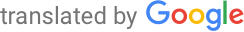Machine-translated page for increased accessibility for English questioners.

# General content and form of entrance exam to NMgr. studies

The entrance exam examines the applicant's ability to successfully study a follow-up master's program at the Faculty of Informatics. It consists of questions from basic knowledge in computer science and mathematics and takes the form of a multi-answer test. Each question gives a choice of one of the five options offered, while always just one is correct. One point is added for the correct answer, 0.25 points are deducted for the incorrect answer; zero points are counted for multiple selected answers or no answer.

#### Thematic areas for the entrance exam:

• Algorithmization and data structures (algorithm complexity, sorting algorithms, basic abstract data structures and their implementation).
• Programming (writing and interpreting a program in a common imperative programming language or pseudocode, principles of object-oriented programming, basic properties of imperative and object-oriented programming languages) and basics of software engineering.
• Database (relational data model, normal forms, SQL, applications).
• Computer networks (unconnected networks, ISO OSI and TCP / IP models, functions, addressing and basic protocols of individual layers, switching and routing in IP network, applications).
• Principles of computer systems (number systems in computer practice, processors, memories, operating system, peripheral devices).
• Graphs and graph algorithms (types of graphs and data structures, distance in graphs, connection of graphs, trees, traversal of graphs, graph skeleton).
• Sets, relations, functions (Cartesian product, potential set, arrangement, equivalence, bijection) and logic (propositional and predicate logic, syntax and semantics, satisfiability, equivalence of formulas).
• Mathematical analysis (analysis of the course of a function, limit, derivation, integral).
• Linear algebra (operations with matrices, linear mappings, solving systems of linear equations).
• Probability and descriptive statistics (elementary combinatorics, conditional probability, distribution of random variables, mean, median, variance, correlation).

#### Evaluation of the entrance exam

Only the answer form is used to evaluate the entrance exam, which is computer scanned and processed anonymously electronically. The answer form is identified by the assigned identification number (application number), which will be sent to the candidate electronically and in a written invitation to the entrance exams. The determining criterion for success in the entrance examinations is the so-called percentile calculated on the basis of the number of correct answers in the entrance examinations and the variant of the entrance examinations that the candidate wrote. The percentile expresses the percentage of applicants who performed less or the same.